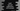# Swift optional explanation with exampleOptional is an important concept in swift. Let’s take an example. In swift, a variable can be defined as below :

``var myString : String = “Hello World !!”``

myString is a String variable with value “Hello World !!” . If you don’t assign any value to this variable anywhere in your code, if you try to set value of ‘myString’ as ‘nil’, it will throw a “compile time error”. That means we will have to assign a non-nil value to a variable always.

## Optional :

Optional is introduced to handle the above problem. In simple word, optional is an enum with two states or Optional type contains either empty value (.None) or some wrapped value (.Some ) . .None/.Some are two states of the enum.

### How to create a swift optional ?

Simple : Just add one ‘?’ after the type name . It will be a optional. Check the below example :

``````var firstValue : Int?
var secondValue : String? = "Hello World !!"

print (firstValue)
print (secondValue)``````

It will print the following output :

``````nil
Optional("Hello World !!”)``````

Both firstValue and secondValue are optionals. for firstValue, we have not assigned any value , so it is holding nil. secondValue is holding wrapped optional value of the “Hello World !!” string.

## Unwrapping an optional value :

In the above example, secondValue is holding one wrapped Optional value, not “Hello World !!” string and to get the value, we need to unwrap it first. We can unwrap an optional value in different ways . Let’s try one by one :

### Forced Unwrapping :

Xcode will always show you one info message to force unwrap an optional value before use. Force unwrap will unwrap the value if it is not nil . If nil, it will throw an error. Try not to use force unwrapping unless you are confident that the value will be non-nil always. To force unwrap, use one ‘!’ sign after the variable name. For the above example, if you want to unwrap secondValue and print :

``````var firstValue : Int?
var secondValue : String? = "Hello World !!"

print (secondValue!)``````

It will print :

``Hello World !!``

But if you try to force unwrap the first value, it will throw one error as its value is nil.

### Optional binding :

Forced unwrapping is not always the best option to unwrap an optional value. You can use it if you are sure that it is not nil in all scenarios. Swift provides another way to unwrap an optional value safely by using a if let statement :

``````var firstValue : Int?
var secondValue : String? = "Hello World !!"

if let first = firstValue{
print("Value of firstValue is \(first)")
}

if let second = secondValue{
print("Value of secondValue is \(second)")
}``````

It will print :

``Value of secondValue is Hello World !!``

It doesn’t execute the print statement of the first if let statement as the value of firstValue is nil.

We can also combine multiple if let statements into one:

``````var firstValue : Int?
var secondValue : String? = "Hello World !!"

if let first = firstValue, let second = secondValue{
print("Value of firstValue is \(first)")
print("Value of secondValue is \(second)")
}``````

It will move inside the if let block only if both unwrapping works. For this example, it will not print anything because firstValue is nil.

### Using guard :

guard is similar to if let. It is used to exit from a block if the unwrapping failed.

``````var firstValue : String?
var secondValue : String? = "Hello World !!"

func getValue(value: String?) -> String{
guard let v = value else{
return "Nil found"
}

return v;
}

print(getValue(value: firstValue))
print(getValue(value: secondValue))``````

In this example, getValue method is used to get the value of an optional String variable. If it is nil, this method returns ‘Nil found’. Else, it returns its value. guard let is used to safely unwrap the optional.

The above example prints the below:

``````Nil found
Hello World !!``````